Sie sind hier: ICP » R. Hilfer » Publikationen

# III.A General Geometric Characterization Theories

A general geometric characterization of porous media should satisfy the following requirements:

• It should be well defined in terms of geometric quantities.

• It should involve only parameters which are directly observable or measurable in an experiment independent of the phenomenon of interest.

• It should not require the specification of too many parameters. The required independent experiments should be simple and economical to carry out. What is economical depends on the available data processing technology. With current data processing technology a characterization requiring more than 1012 numbers must be considered uneconomical.

• The characterization should be usable in exact or approximate solutions of the equations of motion governing the phenomenon of interest.

The following sections discuss methods based on porosities ϕ¯, correlation functions Snr,Cnr, local porosity distributions μϕ, pore size distributions Πr and capacities T𝕂. Table I collects the advantages and disadvantages of these methods according to the specified criteria.

Table I: Advantages and disadvantages of different geometric characterization methods for porous media. A geometrical characterization is called economical if it requires the specification of less than 1012 numbers. ϕ¯, stands for porosity and other numbers defined in section III.A.1. The correlation functions Snr and Cnr are discussed in section III.A.2. The pore size distribution of mercury porosimetry Πr is defined in section III.A.3. The local porosity distributions μϕ are discussed in section III.A.5. The capacities TK are defined in III.A.6.
Characterization well defined predictive economical easily usable
ϕ¯, yes yes yes yes
S2r, yes yes yes yes
Sn(r),(n3) yes yes no yes
Πr no no yes yes
μϕ yes yes yes yes
T𝕂 yes no no no

## III.A.1 Porosity and Other Numbers

### III.A.1.a Porosity

The porosity of a porous medium is its most important geometrical property. Most physical properties are influenced by the porosity.

The porosity ϕ𝕊 of a two component porous medium 𝕊=𝕄 consisting of a pore space (component one) and a matrix space 𝕄 (component two) is defined as the ratio

 ϕ⁢𝕊=V3⁢ℙV3⁢𝕊 (3.1)

which gives the volume fraction of pore space. Here V3 denotes the volume of the pore space defined in (2.3) and V3𝕊 is the total sample volume. In the following the shorthand notation V𝔾=V3𝔾 will often be employed.

The definition (3.1) is readily extended to stochastic porous media. In that case V and ϕ are random variables. If the medium is stationary then one finds using (2.3) and (2.11)

 ϕ = V⁢ℙV⁢𝕊 (3.2) = 1V⁢𝕊⁢∫𝕊χ⁢ℙ⁢r⁢d3⁢r = 1V⁢𝕊⁢∫𝕊χ⁢ℙ⁢r⁢d3⁢r = 1V⁢𝕊∫𝕊Pr{r∈ℙ}d3r = Pr{r0∈ℙ}=⟨χℙ(r0)⟩

where the last line holds only if the medium is stationary. r0 in the last line is an arbitrary point. Although the use of the expectation value from (2.11) requires an underlying discretization a continuous notation was used to indicate that the result holds also in the continuous case. If the stochastic porous medium is not only stationary but also mixing or ergodic, and if it can be thought of as being infinitely extended, then the limit

 ϕ¯=limR⁢𝕊→∞⁡ϕ⁢𝕊=ϕ (3.3)

exists and equals ϕ. Here the diameter R𝔾 of a set 𝔾 is defined as R𝔾=supr1-r2:r1,r2𝔾 as the supremum of the distance between pairs of points. The notation ϕ¯=χr¯ indicates a spatial average while ϕ=χr is a configurational average.

Equation (3.3) represents always an idealization. Geological porous media for example are often heterogeneous on all scales . This means that their composition or volume fraction ϕR𝕊 does not approach a limit for R𝕊. Equation (3.3) assumes the existence of a length scale beyond which fluctuations of the porosity decrease. This scale is used traditionally to define so called “representative elementary volumes” [76, 5]. The problem of macroscopic heterogeneity is related to the remarks in the disccusion of stationarity in section II.B.2. It will be taken up again in section III.A.5 below.

The definition of porosity in (3.1) gives the so called total porosity which has to be distinguished from the open porosity or effective porosity. Open porosity is the ratio of accessible pore volume to total volume. Accessible means connected to the surface of the sample.

The porosity of a simple porous medium 𝕊=𝕄 is related to the bulk density ρ𝕊 the density of the matrix material ρ𝕄 and the density of the pore space material ρ through

 ϕ=ρ⁢𝕄-ρ⁢𝕊ρ⁢𝕄-ρ⁢ℙ. (3.4)

Therefore porosity is conveniently determined from measuring densities using liquid buoyancy or gas expansion porosimetry [3, 1, 77, 2]. Other methods of measuring porosity include small angle neutron, small angle X-ray scattering and quantitative image analysis for total porosity [2, 77, 78, 43, 44]. Open porosity may be obtained from Xylene and water impregnation, liquid metal impregnation, Nitrogen adsorption and air or Helium penetration [77, 44].

Porosity in rocks originates as primary porosity during sedimentation or organogenesis and as secondary porosity at later stages of the geological development . In sedimentary rocks the porosity is further classified as intergranular porosity between grains, intragranular or intercrystalline porosity within grains, fracture porosity caused by mechanical or chemical processes, and cavernous porosity caused by organisms or chemical processes.

### III.A.1.b Specific Internal Surface Area

Similar to the porosity the specific internal surface area is an important geometric characteristic of porous media. In fact, a porous medium may be loosely defined as a medium with a large “surface to volume” ratio. The specific internal surface area is a quantitative measure for the surface to volume ratio. Often this ratio is so large that it has been idealized as infinite [78, 43, 79, 80, 81, 82, 83, 84, 85] and the application of fractal concepts has found much recent attention [58, 86, 87, 88, 84, 42, 89, 90, 91]

The specific internal surface S of a two component porous medium is defined as

 S=V2⁢∂⁡ℙV3⁢𝕊 (3.5)

where V2 is the surface area, defined in eq. (2.3), of the boundary set . The surface area V2 exists only if the internal surface or interface fulfills suitable smoothness requirements. Fractal surfaces would have V2= and in such cases it is necessary to replace the Lebesgue measure in (2.3) with the Hausdorff measure or another suitable measure of the “size” of [58, 59, 60, 61].

The specific internal surface is a characteristic inverse length giving the surface to volume ratio of a porous medium. Typical values for unconsolidated sand are 2×104m-1, and range from 105m-1 to 107m-1 for sandstones [92, 3]. A piece of sandstone measuring 10cm on each side and having a specific internal surface of 107m-1 contains the same area as a sports arena of dimensions 100m×100m. This illustrates the importance of surface effects for all physical properties of porous media.

Specific internal surface area can be measured by similar techniques as porosity. Some commonly employed methods are given in Figure 1 together with their ranges of applicability. Particularly important methods are based on physisorption isotherms [93, 94]. The interpretation of the BET-method  is restricted to certain types of isotherms, and its interpretation requires considerable care. In particular, if micropores are present these will be filled spontaneously and application of the BET-analysis will lead to wrong results . Other methods to determine S measure the two point correlation function. As discussed further in the next section the specific internal surface area can for statistically homogeneous media be deduced from the slope of the correlation function at the origin .

## III.A.2 Correlation Functions

Porosity and specific internal surface area are merely two numbers characterizing the geometric properties of a porous medium. Obviously these two numbers are not sufficient for a full statistical characterization of the system. A full characterization can be given in terms of multipoint correlation functions [96, 97, 98, 99, 100, 101, 102, 103, 104, 6, 105, 106, 107, 108, 109, 110, 111, 112, 8].

The average porosity ϕ of a stationary two component porous medium is given by equation (3.2) as

 ⟨ϕ⟩=⟨χℙ(r0)⟩=Pr{r0∈ℙ} (3.6)

in terms of the expectation value of the random variable χr0 taking the value 1 if the point r0 lies in the pore space and 0 if not. This is an example of a so called one-point function. An example of a two-point function is the covariance function C2r0,r defined as the covariance of two random variables χr and χr0 at two points r0 and r,

 C2⁢r0,r=χ⁢ℙ⁢r0-χ⁢ℙ⁢r0⁢χ⁢ℙ⁢r-χ⁢ℙ⁢r. (3.7)

For a stationary medium the covariance function depends only on the difference r-r0 which allows to set r0=0 without loss of generality. This gives

 C2⁢r=χ⁢ℙ⁢0⁢χ⁢ℙ⁢r-ϕ2. (3.8)

Because χ2r=χr it follows that C20=ϕ1-ϕ. The correlation coefficient of two random variables X and Y is in general defined as the ratio of the covariance covX,Y to the two standard deviations of X and Y [73, 74]. It varies between 1 and -1 corresponding to complete correlation or anticorrelation. The covariance function is often normalized analogous to the correlation coefficient by division with C20 to obtain the two-point correlation function

 G2⁢r=C2⁢rC2⁢0=C2⁢rϕ⁢1-ϕ. (3.9)

An illustration of a two point correlation function can be seen in Figure 14. The porosity in (3.6) is an example of a moment function. The general n-th moment function is defined as

 Sn⁢r1,…,rn=∏i=1nχ⁢ℙ⁢ri (3.10)

where the average is defined in eq. (2.11) with respect to the probability density of microstructures given in eq. (2.10). The covariance function in (3.7) or (3.8) is an example of a cumulant function (also known as Ursell or cluster functions in statistical mechanics). The n-th cumulant function is defined as

 Cn⁢r1,…,rn=∏i=1nχ⁢ℙ⁢ri-χ⁢ℙ⁢ri=∏i=1nχ⁢ℙ⁢ri-ϕ (3.11)

where the second equality assumes stationarity. The cumulant functions are related to the moment functions. For n=1,2,3 one has the relations

 C1⁢r1 = 0 (3.12) C2⁢r1,r2 = S2⁢r1,r2-S1⁢r1⁢S1⁢r2 (3.13) C3⁢r1,r2,r3 = S3⁢r1,r2,r3-S2⁢r1,r2⁢S1⁢r3-S2⁢r1,r3⁢S1⁢r2 (3.14) - S2⁢r2,r3⁢S1⁢r1+2⁢S1⁢r1⁢S1⁢r2⁢S1⁢r3

The analogous moment functions may be defined for the matrix space 𝕄 by replacing χ in all formulas with χ𝕄, and they have been called n-point matrix probability functions [105, 107] or simply correlation functions  From (3.10),(2.10) and (2.11) the probabilistic meaning of the moment functions is found as

 Sn(r1,…,rn)=⟨χℙ(r1)⋅…⋅χℙ(rn)⟩=Pr{r1∈ℙ,…,rn∈ℙ}. (3.15)

Therefore Snr1,,rn is the probability that all the points r1,,rn fall into the pore space.

The case n=2 of the second moment is of particular interest. If the pore space is stationary and isotropic then S2r=S2r and one has S20=ϕ. If the porous medium is also mixing then S2=ϕ2. If the pore space is three dimensional, and does not contain flat twodimensional surfaces of zero thickness then its derivative at the origin is related to the specific internal surface area S through

 S2′⁢0=d⁢S2⁢rd⁢rr=0=-S4. (3.16)

In two dimensions an analogous formula holds in which S is replaced with a “specific internal length” and the denominator 4 is replaced with π.

The practical measurement of two point correlation functions is based on Minkowski addition and subtraction of sets [10, 37]. The Minkowski addition of two sets 𝔸 and 𝔹 in d is defined as the set

 𝔸⊕𝔹=q+r:q∈𝔸,r∈𝔹. (3.17)

Note that 𝔸r=𝔸+r is the translation defined in equation (2.17). Therefore 𝔸𝔹=q𝔹𝔸+q=r𝔸𝔹+r is the union of the translates 𝔹+r as r runs through 𝔸. The dual operation to Minkowski addition is Minkowski subtraction defined as

 𝔸⊖𝔹=⋂q∈𝔹𝔸+q=C⁢C⁢𝔸⊕𝔹 (3.18)

where C𝔸 denotes the complement of 𝔸. With these definitions the two-point function is given as

 S2(r)=Pr{0∈ℙ,r∈ℙ}=Pr{0∈ℙ⊖(-𝔹)} (3.19)

where 𝔹=0,r is the set consisting of the origin and the point r. This formula is the basis for the statistical estimation of S2r and G2r in image analysers from the area of the “eroded” set -𝔹. The operation -𝔹 is called erosion of the set with the set 𝔹 and it has been used in methods to define pore size distributions which will be discussed in the next section. An example for the erosion of a pore space image by a set 0,r is shown in Figure 9.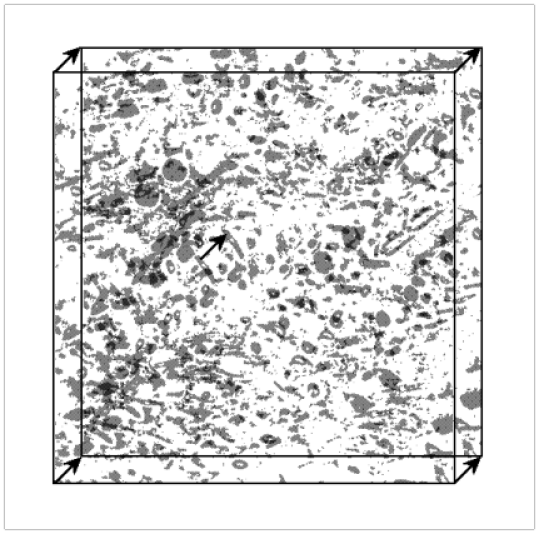Figure 9: Erosion of a pore space image by the set 0,r consisting of the origin 0 and a vector r. The vector -r is shown as an arrow in the image. The overlap of the two images is rendered darker than the rest.

The original image (shown Figure 12) is obtained from a cross section micrograph of a Savonnier oolithic sandstone. Two copies of the image are displaced relative to each other by a vector r as indicated in Figure 9. The two images are rendered in grey, and their intersection is coloured black. The area of the intersection is an estimate for S2r.

The main advantage of the correlation function method for characterizing porous media is that it provides a set of well defined functions of increasing complexity for the geometrical description. In practice one truncates the hierarchy of correlation functions at the two-point functions. While this provides much more information about the geometry than the porosity and specific surface area alone, many important properties of the medium (such as its connectivity) are buried in higher order functions. 2 (This is a footnote:) 2 Two points are called connected if there exists a path between them which lies completely inside the pore space. Therefore the probabilistic description of connectedness properties requires multipoint correlation functions involving all the points which make up the path. Depending on the required accuracy a simple two point function for a three dimensional stationary but anisotropic two component medium could be specified by 106 to 109 data points which would be economical according to the criterion adopted previously. An n point function with the same accuracy would require 106n-1 to 109n-1 data points. Specifying five or higher point functions quickly becomes just as impractical as specifying a given geometry completely.

## III.A.3 “Pore Size” Distributions

In certain porous materials such as wood (see Figure 5) it is natural to identify cylindrically shaped pores and to represent their disorder through a distribution of pore diameters. In other media such as systems with cavernous or oomoldic porosity it is possible to identify roughly convex pore bodies analogous to convex sand grains dispersed in a uniform background. If the radius R of the cylindrical capillaries or spherical pore bodies in such media is randomly distributed then the pore size distribution function Πr can be defined as

 Π(r)=Pr{R≤r} (3.20)

giving the probability that the random radius R of the cylinders or spheres is smaller than r. For general porous microstructures, however, it is difficult to define “pores” or “pore bodies”, and the concept of pore size distribution remains ill defined.

Nevertheless many authors have introduced a variety of well defined probability distributions of length for arbitrary media which intended to overcome the stated difficulty [3, 2, 113, 114, 115, 116, 117, 118, 119]. The concept of pore size distributions enjoys continued popularity in most fields dealing with porous materials. Recent examples can be found in chromatography [120, 121], membranes [122, 123, 124], polymers , ceramics [126, 127, 128, 129], silica gels [130, 131, 132], porous carbon [133, 134], cements [135, 136, 137], rocks and soil science [138, 139, 140, 141, 142, 143], fuel research , separation and adhesion technology [30, 145] or food engineering . The main reasons for this popularity are adsorption measurements [147, 30, 148] and mercury porosimetry [149, 150, 151, 152].

### III.A.3.a Mercury Porosimetry

The “pore size distribution” of mercury porosimetry is not a geometric but a physical characteristic of a porous medium. Mercury porosimetry is a transport and relaxation phenomenon [43, 153], and its discussion would find a more appropriate place in chapter V below. On the other hand “pore size distributions” are routinely measured in practice using mercury porosimetry, and many readers will expect its discussion in a section on pore size distributions. Therefore pore size distributions from mercury porosimetry are discussed already here together with other definitions of this important concept.

Mercury porosimetry is based on the fact that mercury is a strongly nonwetting liquid on most substrates, and that it has a high surface tension. To measure the “pore size distribution” a porous sample 𝕊 with pore space is evacuated inside a pycnometer pressure chamber at elevated temperatures and low pressures . Subsequently the sample is immersed into mercury and an external pressure is applied. As the pressure is increased mercury is injected into the pore space occupying a subset HgP of the pore space which depends on the applied external pressure P. The experimenter records the injected volume of mercury VHgP as a function of the applied external pressure. If the volume of the pore space V is known independently then this gives the saturation SHgP=VHgP/V as a function of pressure. The cumulative “pore size” distribution function ΠHg(r)=Pr{Rr} of mercury porosimetry is now defined by

 ΠHg⁢r=1-SHg⁢2⁢σHg⁢cos⁡θr. (3.21)

For rocks a contact angle θHg135 and surface tension with vacuum of σHg0.48Nm-1 are commonly used [1, 43, 153]. The definition of ΠHgr is based on the equation

 Pc⁢a⁢p=2⁢σHg⁢cos⁡θr (3.22)

for the capillary pressure Pcap which expresses the force balance in a single cylindrcal capillary tube. Equation (3.21) follows from (3.22) if it is assumed that the saturation history SHgP is identical to that obtained from the so called capillary tube model discussed in section III.B.1 below. The capillary tube model is a hypothetical porous medium consisting of parallel nonintersecting cylindrical capillaries of random diameter.

The fact that ΠHgr is not a geometrical quantity but a capillary pressure function is obvious from its definition. It depends on physical properties such as the nature of the injected fluid or wetting properties of the walls. For a suitable choice of tube diameter the function SHgP of pressure could equally well be translated into a distribution of the wetting angles θ. SHgp shows hysteresis implying that the pore size distribution ΠHgr is process dependent.

Although ΠHgr is not a geometrical quantity it contains much useful information about the microstructure of the porous sample. An example for the information obtained from mercury porosimetry is shown in Figure 10 together with an image of the rock for which it was measured .3 (This is a footnote:) 31 MPa = 10 bar = 107 dyn/cm2 = 9.869 atm = 145.04 psi The rock is an example for a medium with hollow pores. The correct interpretation of the saturation history SHgp obtained from mercury porosimetry continues to be an active research topic [154, 155, 156, 157, 158, 159, 160].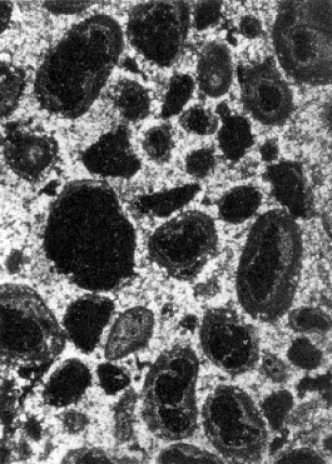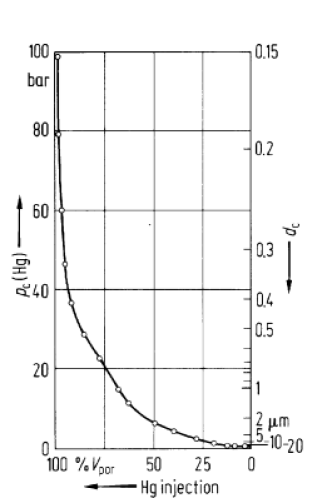Figure 10: a) Crossed nicols image of Dolomite oncolithe. The image width corresponds to 2.3 mm. The porosity is 11%, the permeability 11 md. b) Capillary presssure curve for the rock shown in the image. The right axis shows the ”pore size” scale. [Reproduced with permission from . Copyright Springer Verlag 1982.]

### III.A.3.b Random Point Methods

Several authors [3, 113, 116] suggest to define the “pore size” by first choosing a point r at random in the pore space, then to choose a compact set 𝕂 containing r, and finally to enlarge 𝕂 until it first intersects the matrix space 𝕄. In its simplest version [3, 116] the set 𝕂 is chosen as a small sphere 𝔹r,ϵ of radius ϵ (see (2.5) above). Then the pore size distribution Πrpx of the random point method is defined as the distribution function Πrp(x)=Pr{R(r)x} of the random variable Rr defined as

 R⁢r=inf⁡ϵ:𝔹⁢r,ϵ∩𝕄≠∅. (3.23)

Here R is a random variable because r is chosen at random.

In a more sophisticated version of the same idea the set 𝕂 is chosen as a small coordinate cross whose axes are then increased independently until they first touch the matrix space. [113, 2]. This gives direction dependent pore size distributions.

The main weakness of such a definition is that it is imprecise. This becomes apparent from the fact that the randomness of the pore sizes Rr does not arise from the irregularities of the pore space, but from the random placement of r. Consider a regular pore space consisting of nonintersecting spheres of equal radii R0 centered at the vertices of a simple (hyper)cubic lattice. Assuming that the points r are chosen at random with a uniform distribution it follows that the pore size distribution Πrp is not given by a δ-function at R0 but instead as a uniform distribution on the interval 0,R0. More dramatically, exactly the same pore size distribution is obtained for every pore space made from nonintersecting spheres of equal radii, no matter whether they are placed randomly or not. In addition Πrpx can be changed arbitrarily by changing the distribution function governing the random placement of r.

A more precise formulation of the same idea is possible in terms of conditional probabilities. In section III.A.4 it will be seen that the vague ideas underlying random point methods are given a precise definition in the form of contact and chord length distributions [10, 37]

### III.A.3.c Erosion Methods

Another approach to the definition of a geometrical pore size distribution [117, 118, 119] is borrowed from the erosion operation in image processing [161, 162]. Erosion is defined in terms of Minkowski addition and subtraction of sets introduced above in (3.17) and (3.18). The erosion of a set 𝔸 by a set 𝔹 is defined as the map 𝔸𝔸-𝔹=q𝔹𝔸-q. The erosion was illustrated in Figure 9 for a pore space image and a set 𝔹=0,r.

The method for locating “pore chambers”, “pore channels” and “pore throats” suggested in  is based on eroding the matrix space 𝕄 of a two component porous medium. A ball 𝔹0,ϵ is chosen as the structuring element. The erosion 𝕄-𝔹0,ϵ shrinks the matrix space 𝕄. The erosion operation is repeated until the matrix space decomposes into disconnected fragments. Continuing the erosion the pieces may either fragment again or become convex. If a piece becomes convex it is called a “grain”. The centroid of the conves grain is called a “grain center”. Reversing the erosion process allows to locate the point of first/last contact of two fragments. Connecting neighbouring grain centers by a path through their last contact point produces a network model of the grain space. Having defined a network of grain centers and last contact points the authors of , and their followers [119, 118], suggest to erect contact “surfaces” in each contact point. A contact plane is defined as a “minimum area cross section” of 𝕄. Subsequently a ball is placed at each grain center and continually enlarged. When the enlargement encounters a surface plane the ball is truncated at the surface plane and only its non-truncated pieces continue to grow until the sample space is completely filled with the inflated grains. The intersection points of three or more planes in the resulting tesselation of space are defined to be pore chambers. The intersection lines of two planes are called pore channels, and “minimal-area cross sections” of the pore space along the pore channels are called pore throats. The pore throats are not unique, and sensitive to details of the local geometry. The pore chambers and the pore channels will in general not lie in the pore space.

A drawback of this procedure is that it is less unique than it seems at first sight. The network constructed from eroding the pore space is not unique because the erosion operation involves the set 𝔹 as a structuring element, and hence there are infinitely many erosions possible. The resulting grain network depends on the choice of the set 𝔹, a fact which is not discussed in [117, 119]. Figure 11 shows an example where erosion with a sphere produces three grains while erosion with an ellipsoid produces only two grains. The original set consists of the grey and black region, the eroded part is coloured grey, and the residual set is coloured black. The theoretically described procedure for determining the network was not carried out in practice . Instead “subjective human preprocessing” (,p.4158) was used to determine the network.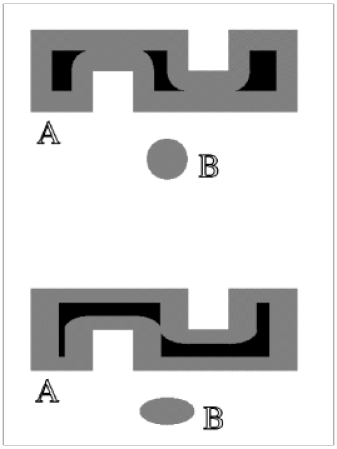Figure 11: Erosion 𝔸-𝔹 of a set 𝔸 by a set 𝔹 for the cases where 𝔹 is a sphere or an ellipsoid. For a sphere the eroded set (shown in black) has three components while for an ellipsoid it consists of only two connected components.

The hydraulic radius method [114, 115, 2] for determining pore size distributions is based on the idea of “symbolically closing pore throats”. The definition of pore throats is given in terms of “cross sections” of the pore space. A cross section could be defined as the intersection of a plane 𝔼q,r0, characterized by its unit normal q𝔹0,1 and a point r0 in the plane, with the pore space and some suitable set 𝔾q,r0 which represents the region of interest and could depend on the choice of plane. In symbols q,r0=𝔼q,r0𝔾q,r0. A pore throat containing the point r0 is then defined as

 ℂ*⁢r0=minq∈∂⁡𝔹⁢0,1⁡V2⁢ℂ⁢q,r0V1⁢∂⁡ℂ⁢q,r0 (3.24)

where the minimum is taken over the unit sphere of orientations of the planes. A pore throat is now defined as a local minimum of the function *r0 as r0 is varied over the pore space. This ideal definition has in practice been replaced with a subjective choice of orientations based on the assumption of isotropy [114, 115, 2]. After constructing all pore throats of a medium the pore space becomes divided into separate compartments called “pore bodies” whose “size” can then be measured by a suitable measure such as the volume to the power 1/3.

The definition of pore throats in hydraulic radius methods is very sensitive to surface roughness. This is readily seen from an idealized spherical pore with a few spikes. Another problem as remarked in , page 586, is that “the size of a pore body is not readily related in a unique manner to any measurable physical quantity”.

## III.A.4 Contact and Chord Length Distributions

Chord length distributions [163, 164, 165, 166] are special cases of so called contact distributions [10, 37]. Consider the random matrix space 𝕄 of a two component stochastic porous medium and choose a compact set 𝕂 containing the origin 0. Then the contact distribution is defined as the conditional probability

 Π𝕂⁢x = 1-Pr{0∉𝕄⊕(-x𝕂))|0∉𝕄} (3.25) = 1-Pr{0∉𝕄⊕(-x𝕂)}1-ϕ

for x0. Here ϕ denotes the bulk porosity as usual. Two special choices of the compact set 𝕂 are of particular importance. These are the unit sphere 𝕂=𝔹0,13 and the the unit interval 𝕂=0,1.

For a unit sphere 𝕂=𝔹0,1 the quantity 1-Π𝔹0,1x is the conditional probability that a fixed point in the pore space is the center of a sphere of radius x contained completely in the pore space, under the condition that the chosen point does not belong to 𝕄. If 𝕄 is isotropic and its boundary is sufficiently smooth then the specific internal surface S can be obtained from the derivative at the origin as 

 S=ϕ⁢dd⁢x⁢Π𝔹⁢0,1⁢xx=0. (3.26)

The spherical contact distribution Π𝔹0,1x provides a more precise formulation of the random point generation methods for pore size distributions [3, 116] discussed in subsection III.A.3.b.

For the unit interval 𝕂=0,1 the contact distribution Π0,1x is related to the chord length distribution Πclx giving the probability that an interval in the intersection of with a straight line containing the unit interval has a length smaller than x. This provides a more precise formulation of the random point generation ideas in [113, 2]. The relation between the contact distribution and the chord length distribution is given by the equation

 Π0,1⁢x=1-∫x∞y-x⁢d⁢Πc⁢l⁢y∫0∞y⁢d⁢Πc⁢l⁢y (3.27)

where the denominator on the right hand side gives the mean chord length ¯=0ydΠcly. The mean chord length is related to the specific internal surface area through ¯=4ϕ/S. In section III.A.2 it was mentioned that the specific internal surface area can be obtained from the two point function (see 3.16). Therefore also the mean chord length can be related to the correlation function through

 ℓ¯=-ϕS2′⁢0 (3.28)

Along these lines it has been suggested in  that the full chord length distribution can be obtained directly from small angle scattering experiments.

Contact and chord length distributions provide much more geometrical information about the porous medium than the porosity and specific surface area, and are at the same time not as unnecessarily detailed as the complete specification of the deterministic or stochastic geometry. Depending on the demands on accuracy a contact or chord length distribution may be specified by 10 to 1000 numbers irrespective of the microscopic resolution. This should be compared with 1024 or 21024 numbers for a full deterministic or stochastic characterization.

## III.A.5 Local Geometry Distributions

### III.A.5.a Local Porosity Distribution

Local porosity distributions, or more generally local geometry distributions, provide a well defined general geometric characterization of stochastic porous media. [168, 169, 170, 171, 172, 173, 174, 175]. Local porosity distributions were mainly developed as an alternative to pore size distributions (see section III.A.3). They are intimately related with the theory of finite size scaling in statistical physics [176, 177, 64, 178]. Although fluctuations in the porosities have been frequently discussed [179, 10, 37, 2, 5, 180, 181, 76, 182], the concept of local porosity distributions and its relation with correlation functions was developed only recently [168, 169, 170, 171, 172, 173, 174, 175]. More applications are being developed [183, 184].

Local porosity distributions can be defined for deterministic as well as for stochastic porous media. For a single deterministic porous medium consider a partitioning 𝒦=𝕂1,,𝕂M of the sample space 𝕊 into M mutually disjoint subsets, called measurement cells 𝕂j. Thus j=1M𝕂j=𝕊 and 𝕂i𝕂j= if ij. A particular partitioning was used in the orginal publications [178, 169, 170, 171] where the 𝕂j are unit cells centered at the vertices of a Bravais lattice superimposed on 𝕊. This has the convenient feature that the 𝕂j are translated copies of one and the same set, and they all have the same shape. An example is illustrated in Figure 12 showing a quadratic lattice as the measurement grid in two dimensions superposed on a thin section of an oolithic sandstone.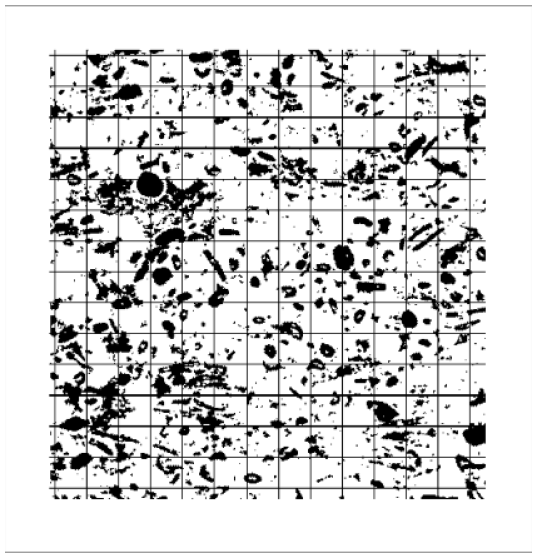Figure 12: Measurement lattice of squares superimposed on the discretized thin section image of a sandstone. The pore space is coloured black, the matrix space is rendered white.

The local porosity inside a measurement cell 𝕂j is defined as

 ϕ⁢𝕂j=V⁢ℙ∩𝕂jV⁢𝕂j=1Mj⁢∑ri∈𝕂jχ⁢ℙ⁢ri (3.29)

where the second equality applies in case of discretized space and Mj denotes the number of volume elements or voxels in 𝕂j. Thus the empirical one cell local porosity density function is defined as

 μ~⁢ϕ;𝒦=1M⁢∑j=1Mδ⁢ϕ-ϕ⁢𝕂j (3.30)

where δx is the Dirac δ-distribution. Obviously the distribution depends on the choice of partitioning the sample space. Two extreme partitions are of immediate interest. The first arises from setting M=N and thus each 𝕂j contains only one individual volume element 𝕂j=rj with 1jM=N. In this case ϕ𝕂j=0 or ϕ𝕂j=1 depending on whether the volume element falls into matrix space (0), or pore space (1). This gives immediately

 μ~⁢ϕ;r1,…,rN=ϕ⁢𝕊⁢δ⁢ϕ-1+1-ϕ⁢𝕊⁢δ⁢ϕ (3.31)

where ϕ𝕊 is the total porosity. The other extreme arises for M=1 and thus 𝕂1=𝕊 the measurment cell coincides with the sample space. In this case obviously

 μ~⁢ϕ;𝕊=δ⁢ϕ-ϕ⁢𝕊. (3.32)

Note that in both extreme cases the local porosity density is completely determined by the total porosity ϕ𝕊, which equals ϕ𝕊=ϕ¯ if the sample is sufficiently large and mixing or ergodicity (3.3) holds.

For a stochastic porous medium the one cell local porosity density function is defined for each measurement cell as

 μ⁢ϕ;𝕂j=δ⁢ϕ-ϕ⁢𝕂j (3.33)

where 𝕂j𝒦 is an element of the partitioning of the sample space. For the finest partition with M=N and 𝕂j=rj one finds now using eq. (3.2)

 μ⁢ϕ;rj = Pr{X(rj)=1}δ(ϕ-1)+Pr{X(rj)=0}δ(ϕ) (3.34) = ϕ⁢δ⁢ϕ-1+1-ϕ⁢δ⁢ϕ

independent of j. If mixing (3.3) holds then ϕ𝕊=ϕ=ϕ¯ if the sample becomes sufficiently large, and the result becomes identical to equation (3.31) for deterministic media. In the other extreme of the coarsest partition one finds

 μ⁢ϕ;𝕊=δ⁢ϕ-ϕ⁢𝕊 (3.35)

which may in general differ from (3.32) even if the sample becomes sufficiently large, and mixing holds. This is an important observation because it emphasizes the necessity to consider more carefully the infinite volume limit 𝕊d.

If a large deterministic porous medium is just a realization of a stochastic medium obeying the mixing property, and if the 𝕂j are chosen such that the random variables ϕ𝕂j are independent, then Gliwenkos theorem  of mathematical statistics guarantees that the empirical one cell distribution approaches μϕ;𝕂 in the limit M. In symbols

 limM→∞⁡μ~⁢ϕ;𝒦=μ⁢ϕ;𝕂j (3.36)

where the right hand side is independent of the choice of 𝕂j. Therefore μ and μ~ are identified in the following. This identification emerges also from considering average and variance as shown next.

Define the average local porosity ϕ¯=01ϕμϕdϕ as the first moment of the local porosity distribution. For a stationary (=homogeneous) porous medium the definitions (3.33) and (3.29) immediately yield

 ϕ⁢𝕂j¯ = ∫01ϕ⁢μ⁢ϕ;𝕂j⁢d⁢ϕ (3.37) = ϕ⁢𝕂j = 1Mj⁢∑ri∈𝕂jχ⁢ℙ⁢ri = ϕ

where 𝕂j is a measurement cell. Similarly, the variance of local porosities reads

 ϕ⁢𝕂j-ϕ⁢𝕂j¯2¯ = ∫01ϕ-ϕ⁢𝕂j¯2⁢μ⁢ϕ;𝕂j⁢d⁢ϕ (3.38) = ∫01ϕ2⁢δ⁢ϕ-ϕ⁢𝕂j⁢d⁢ϕ-ϕ¯2 = ϕ⁢𝕂j2-ϕ⁢𝕂j2 = 1Mj2⟨[Mjϕ-∑i=1Mjχℙ(ri)]⟩2 = ϕMj⁢1-ϕ+2Mj2⁢∑i≠kri,rk∈𝕂jC2⁢ri-rk.

This result is important for two reasons. Firstly it relates local porosity distributions to correlation functions discussed above in section III.A.2. Secondly it shows that the variance depends inversely on the volume Mj of the measurement cell. This observation reconciles eq. (3.35) for stochastic media with eq. (3.32) for deterministic media because it shows that μϕ;𝕂j approaches a degenerate δ-distribution in the limit Mj. Together with (3.37) and Mj=N and M=1 for 𝕂j=𝕊 this shows that (3.35) and (3.32) become equivalent.

The n-cell local porosity density function μnϕ1,,ϕn;𝕂1,,𝕂n is the probability density to find local porosity ϕ1 in measurement cell 𝕂1, ϕ2 in 𝕂2 and so on until n. Formally it is defined by generalizing (3.33) to read

 μn⁢ϕ1,…,ϕn;𝕂1,…,𝕂n=δ⁢ϕ1-ϕ⁢𝕂1⋅…⁢δ⁢ϕn-ϕ⁢𝕂n (3.39)

where the sets 𝕂1,,𝕂n𝒦 are a subset of measurement cells in the partition 𝒦. Note that the n-cell functions μn are only defined for M>n, i.e. if there are sufficient number of cells. In particular for the extreme case M=1 only the one-cell function is defined. In the extreme case M=N of highest resolution (𝕂j=rj) the moments of the n-cell local porosity distribution reproduce the moment functions (3.10) of the correlation function approach (section III.A.2) as

 ϕi1⋅…⋅ϕim¯ = ∫01…⁢∫01ϕi1⋅…⋅ϕim⁢μn⁢ϕ1,…,ϕn;r1,…,rn⁢d⁢ϕ1⁢…⁢d⁢ϕn (3.40) = χ⁢ℙ⁢ri1⋅…⋅χ⁢ℙ⁢rim = Sm⁢ri1,…,rim

where mn must be fulfilled. This provides a connection between local porosity approaches and correlation function approaches. Simultaneously it shows that the local porosity distributions are significantly more general than correlation functions. Already the one-cell function μ contains more information than the two-point correlation functions S2 or C2 as demonstrated on test images in .

The most important practical aspect of local porosity distributions is that they are easily measurable in an independent experiment. Experimental determinations of local porosity distributions have been reported in [186, 173, 174, 175]. They were obtained from two dimensional sections through a sample of sintered glass beads. An example from  is shown in Figure 13.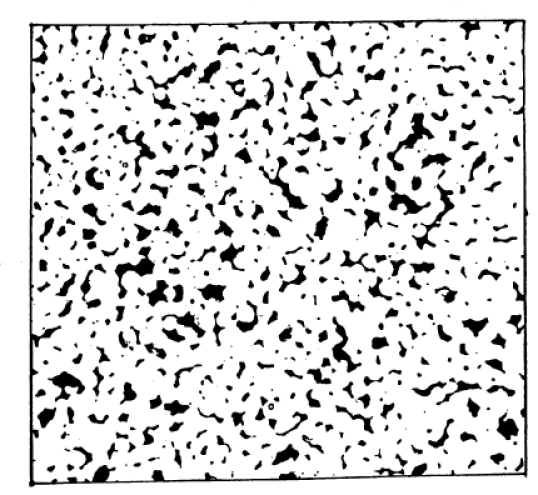Figure 13: Pore space image of sintered 250μm glass beads with total porosity of 10.7%. The pore space is rendered in black, the matrix space in white. 

The porousmedium was made from sintering glass beads of roughly 250μm diameter. Scanning electron migrographs obtained from the specimen were then digitized with a spatial resolution of 4.1μm per pixel. The pore space is represented black in Figure 13 and the total porosity is ϕ¯=10.7%. The pixel-pixel correlation function of the pore space image is shown in Figure 14.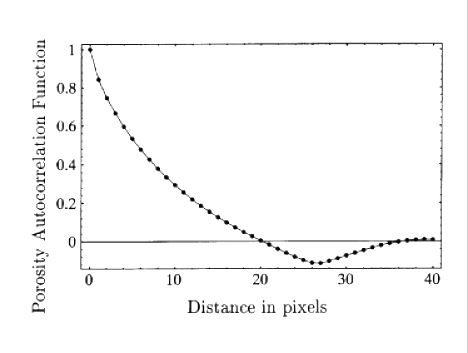Figure 14: Pixel-pixel porosity correlation function G2r for the image shown in Figure 13. The distance r is measured in pixels corresponding to 4.1μm per pixel .

The local porosity distribution was then measured using a square lattice with lattice constant L. The results are shown in Figure 15 for measurement cell sizes of L=40,50,60 and L=80 pixels.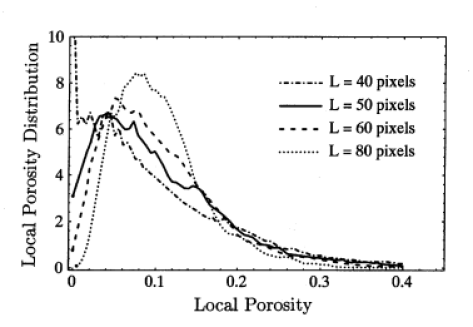Figure 15: Local porosity probability density function μϕ;L measured from square shaped measurement cells from the image shown in Figure 13. The dash-dotted line corresponds to L=40 pixels, the solid line to L=50, the dashed line to L=60 and the dotted line to L=80 pixels. One pixels corresponds to 4.1μm. 

Measurements of the local porosity distribution from three dimensional pore space images are currently carried out . The pore space is reconstructed from serial sections which is a standard, but costly, technique to obtain threedimensional pore space representations [162, 187, 117, 113, 188, 114, 2, 183]. The advent of synchrotron microtomography [119, 4] and laser scanning confocal microscopy [188a] promises to reduce the cost and effort.

### III.A.5.b Local Geometry Entropies

The linear extension L of the measurement cells is the length scale at which the pore space geometry is described. The length L can be taken as the side length of a hypercubic measurement cell, or more generally as the diameter R𝕂=supr1-r2:r1,r2𝕂 of a cell 𝕂 defined as the supremum of the distance between pairs of points. As L the local porosity distribution approaches a δ-distribution concentrated at ϕ¯ according to (3.32) and (3.35). For l0 on the other hand it approaches two δ-distributions concentrated at 0 and 1 according to (3.31) and (3.34). In both limits the local porosity distribution contains only the bulk porosity ϕ¯=ϕ=ϕ𝕊 as a geometric parameter. At intermediate scales the distribution contains additional information, such as the variance of the porosity fluctuations. This suggests to search for an intermediate scale L* which provides an optimal description. Several criteria for determining L* were discussed in . One interesting possibility is to optimize an information measure or entropy associated with μϕ;L, and to define the entropy function

 I⁢L=∫01μ⁢ϕ;L⁢log⁡μ⁢ϕ;L⁢d⁢ϕ (3.41)

relative to the conventional a priori uniform distribution. The so called entropy length is then obtained from the extremality condition

 d⁢μ⁢ϕ;Ld⁢LL=L*=0. (3.42)

That the entropy length L* exists and is well defined was first demonstrated in  using synthetic computer generated images. Figure 16 shows the function IL calculated for the image displayed in Figure 13. A clear minimum appears at L40 pixels corresponding to 164μm.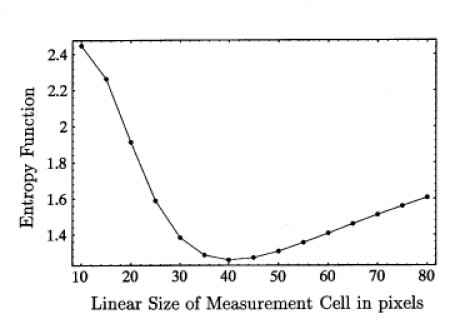Figure 16: Entropy function as a function of the linear size of the measurement cell in pixels for the local porosity probability density functions obtained from the image shown in Figure 13. One pixel corresponds to 4.1μm.

A similar entropy analysis was recently discussed in  for thin film morphologies of composites. The entropy function H*L defined in  is not equivalent to IL above because it is not additive. Nevertheless it was recently found  that H*L and IL give the same value of L*.

### III.A.5.c Local Specific Internal Surface Distributions

Local specific internal surface area distributions are a natural generalization of local porosity ditributions which was first introduced in  in the study of fluid transport in porous media. Define the local specific internal surface area in a cell 𝕂j as

 S⁢𝕂j=V2⁢∂⁡ℙ∩𝕂jV3⁢𝕂j=1Mj⁢∑ri∈𝕂jχ⁢∂⁡ℙ⁢ri (3.43)

which is analogous to equation (3.29). Generalizing equations (3.30) and (3.33) the local specific internal surface area probability density is defined as

 μ⁢S;𝕂j=δ⁢S-S⁢𝕂j. (3.44)

in analogy with equation (3.33). The joint probability density μϕ,S;𝕂j to find a local porosity ϕ and local specific internal surface area in the range ϕ to ϕ+dϕ and S to S+dS will be called local geometry distribution and it is defined as the probability density

 μ⁢ϕ,S;𝕂j=δ⁢ϕ-ϕ⁢𝕂j⁢δ⁢S-S⁢𝕂j. (3.45)

The average specific internal surface area in a measurement region 𝕂 is then obtained from the local geometry distribution as

 S⁢𝕂¯=∫0∞∫0∞S⁢μ⁢ϕ,S;𝕂⁢d⁢ϕ⁢d⁢S (3.46)

and it represents an important local length scale.

Of course local geometry distributions can be extended to include other well defined geometric characteristics such as mean curvature or topological invariants. The definition of the generalized local geometry distribution is then obtained by generalizing (3.45).

### III.A.5.d Local Percolation Probability

In addition to the local porosity distributions and local specific internal surface area distributions it is necessary to characterize the geometrical connectivity properties of a porous medium. This is important for discussing transport properties which depend critically on the connectedness of the pore space, but are less sensitive to its overall porosity or specific internal surface.

Two points inside the pore space of a two component porous medium are called connected if there exists a path contained entirely within the pore space which connects the two points. Using this connectivity criterion a cubic measurement cell 𝕂 is called percolating if there exist two points on opposite surfaces of the cell which are connected to each other. The local percolation probability λϕ,S;𝕂 is defined as the probability to find a percolating geometry in measurement cells 𝕂 whose local porosity is ϕ and whose local specific internal surface area is S. In practice the estimator for λϕ,S;𝕂 is the fraction of percolating measurement cells which have the prescribed values of ϕ and S.

The average local percolation probability defines an important global geometric characteristic

 p(𝕂)=∫0∞∫01λ(ϕ,S,;𝕂)μ(ϕ,S;𝕂)dϕdS (3.47)

which gives the total fraction of percolating local geometries. It will be seen in section V.B.4 and V.C.6 that p𝕂 is an important control parameter for the connectivity of the porous medium.

### III.A.5.e Large Scale Local Porosity Distributions

This section reviews the application of recent results in statistical physics [190, 63, 64, 65, 178, 66, 67, 68, 69] to the problem of describing the macroscopic heterogeneity on all scales. The original definition (3.33) of the local porosity distributions depends upon the size and shape of the measurement cells, respectively on the partitioning 𝒦 of the sample space. This dependence on the choice of a test set or “structuring element” is characteristic for many methods of mathematical morphology [10, 37, 71], and many of those discussed in sections III.A.3 and III.A.4 above. On the other hand subsection III.A.5.a has shown that in the limit of large measurement cells R𝕂 the form of the local porosity distribution μϕ;𝕂 becomes independent of 𝕂 and approaches one and the same universal limit given by δϕ-ϕ¯. This behaviour is an expression of the central limit theorem. Local porosity distributions have support in the unit interval, hence their second moment is always finite, and average local porosities must become sharp in the limit. It will be seen now that this behaviour is indeed characteristic for macroscopically homogeneous porous media, while other limiting distributions may arise for macroscopically heterogeneous media.

Consider a convex measurement cell 𝕂 of volume V0. Let b be the random scale factor at which the pore space volume Vb𝕂 of the inflated measurement cell b𝕂 first exceeds V0, i.e. define b as

 b=inf⁡c≥1:V⁢ℙ∩c⁢𝕂≥V0. (3.48)

Consider N mutually disjoint measurement cells 𝕂i 1iN all having the same volume V𝕂i=V0. Let bi denote the scale factors associated with the cells, and let Vi=Vbi𝕂i be the N values of the pore space volumes. If the medium is homogeneous there exists a finite correlation length beyond which fluctuations decrease. Then the nonoverlapping cells 𝕂i can be chosen such that the inflated cells remain nonoverlapping, and such that they are separated more than the correlation length. Then the N local porosities ϕ𝕂i=V0/Vi are uncorrelated random variables. For macroscopically heterogeneous media the correlation length may be infinite, and thus it is necessary to consider the limit V0 of infinitely large cells to obtain uncorrelated porosities. In [190, 63, 64, 65, 178] the resulting ensemble limit N,V0 has been defined and studied in detail. In the present context the ensemble limit can be used to study the limiting distribution of the N-cell porosity

 ϕ⁢𝕂1,…,𝕂N=N⁢V0V1+…+VN=N⁢∑i=1Nϕ⁢𝕂i-1-1 (3.49)

obtained from the N measurements. In the ensemble limit the N porosities ϕ𝕂i become independent but ill defined, and this suggests to consider instead the limiting behaviour of the renormalized sums of positive random variables

 φN=1DN⁢∑i=1Nϕ⁢𝕂i-1-CN (3.50)

where DN>0 and CN are renormalization constants. Note that ϕ𝕂i-11 for all i.

If the sequence of distribution functions of the random variables φN converges in the ensemble limit N and V0 then the limiting distribution is given by a stable law . The existence of this limit is an indication of fractional stationarity [63, 64, 65, 178, 68, 69]. The limiting probability density function for the variables φN is obtained along the lines of  as

 h(φ;ϖ,C,D)=1ϖ⁢φ-CH1110(D1/ϖφ-C|0,10,1/ϖ) (3.51)

where φC and the parameters obey the restrictions 0<ϖ<1, D0 and C1. The function H1110 appearing on the left hand side is a generalized hypergeometric function which can be defined through a Mellin-Barnes contour integral . The limiting local porosity density is obtained as the distribution of the random variable ϕ=1/φ, and reads

 μ(ϕ;ϖ,C,D)=1ϖ⁢ϕ⁢1-C⁢ϕH1110(D1/ϖ⁢ϕ1-C⁢ϕ|0,10,1/ϖ) (3.52)

for 0ϕ1/C and μϕ=0 for ϕ>1/C. The universal limiting local porosity distributions μϕ;ϖ,C,D depend on only three parameters, and are independent of the diameter or size of the measurement cells 𝕂i. It is plausible that the limiting distributions will also be independent of the shape of the measurement cells, at least for the classes and sequences of convex measurement sets usually employed in studying the thermodynamic limit .

Because the limiting distributions have their support in the interval 0ϕ1/C all its moment exist, and one has ϕn¯=ϕn¯ϖ,C,D. If the moments for n=1,2,3 can be inverted then the parameters ϖ,C,D can be written as

 ϖ=ϖ⁢ϕ¯,ϕ2¯,ϕ3¯,C=C⁢ϕ¯,ϕ2¯,ϕ3¯⁢D=D⁢ϕ¯,ϕ2¯,ϕ3¯ (3.53)

in terms of the first three integer moments. Figure 17 displays the form of μϕ;1/2,2,D for various values of D.Figure 17: Universal limiting local porosity density (3.52) for ϖ=0.5,C=2 and D=0.05,0.5,1.0,2.0,5.0,10.0. For D0 the density function is concentrated at ϕ=1/C, and it vanishes generally for ϕ>1/C.

In the limit of small porosities ϕ0 the result (3.52) behaves as a power law

 μ⁢ϕ∝ϕϖ-1. (3.54)

Within local porosity theory this behaviour can give rise to scaling laws in transport and relaxation properties of porous media . The importance of universal limiting local geometry distributions arises from the fact that there exists a class of limit laws which remains broad even after taking the macroscopic limit N,V0. This is the signature of macroscopic heterogeneity, and it occurs for ϖ<1. Macroscopically homogeneous systems, corresponding to ϖ=1, converge instead towards a δ-distribution concentrated at the bulk porosity ϕ¯.

## III.A.6 Capacities

While local porosity distributions (in their one cell form) give a useful practical characterization of stochastic porous media they do not characterize the medium completely. A complete characterization of a stochastic medium is given by the so called Choquet capacities [72, 10]. Although this characterization is very important for theoretical and conceptual purposes it is not practical because it requires to specify the set of “all” compact subsets (see discussion in section II.B.2).

Consider the pore space of a stochastic two component porous medium as a random set. Let denote the family of all closed sets and 𝒦 the set of all compact sets as in section II.B.2 above. For any 𝕂𝒦 the “hitting function” or capacity functional is defined as

 T(𝕂)=Pr{ℱ𝕂}=Pr{ℙ∩𝕂≠∅} (3.55)

where Pr is the probability law governing the random set . Then T has the following properties: (i) T=0 and 0T𝕂1. (ii) If 𝕂1,,𝕂n, is a sequence of compact subsset then 𝕂1𝕂 implies T𝕂n𝕂. (iii) For all n the numbers Sn𝕂;𝕂1,,𝕂n0 are nonnegative where the Sn are determined by the recursion relation

 Sn⁢𝕂;𝕂1,…,𝕂n=Sn-1⁢𝕂;𝕂1,…,𝕂n-1-Sn-1⁢𝕂∪𝕂n;𝕂1,…,𝕂n-1 (3.56)

and S0𝕂=1-T𝕂. The number Sn𝕂;𝕂1,,𝕂n gives the probability that 𝕂 is empty, but 𝕂i is not empty for all i. The functional T𝕂 is called an alternating Choquet capacity of infinite order[10, 71].

Choquet’s theorem says that the converse is also true. Explicitly, if T is a functional on 𝒦, then there exists a necessarily unique distribution Pr in 𝔉 with

 Pr⁢ℱ𝕂=T⁢𝕂 (3.57)

for all 𝕂𝒦 if and only if T is an alternating Choquet capacity of infinite order. This theorem shows that capacity functionals play the same defining role for random sets in continuous space as do the numbers μx1,,xN in (2.10) in the discrete case.

The main problem with this theoretically important result is that the family of hitting sets ℱ𝕂 is much too large for both practical and theoretical purposes.